# NCERT Solutions For Class 10 Maths Chapter 6 Exercise 6.1 – Triangles

Download NCERT Solutions For Class 10 Maths Chapter 6 Exercise 6.1 – Triangles. This Exercise contains 3 questions, for which detailed answers have been provided in this note. In case you are looking at studying the remaining Exercise for Class 10 for Maths NCERT solutions for other Chapters, you can click the link at the end of this Note.

### NCERT Solutions For Class 10 Maths Chapter 6 Exercise 6.1 – Triangles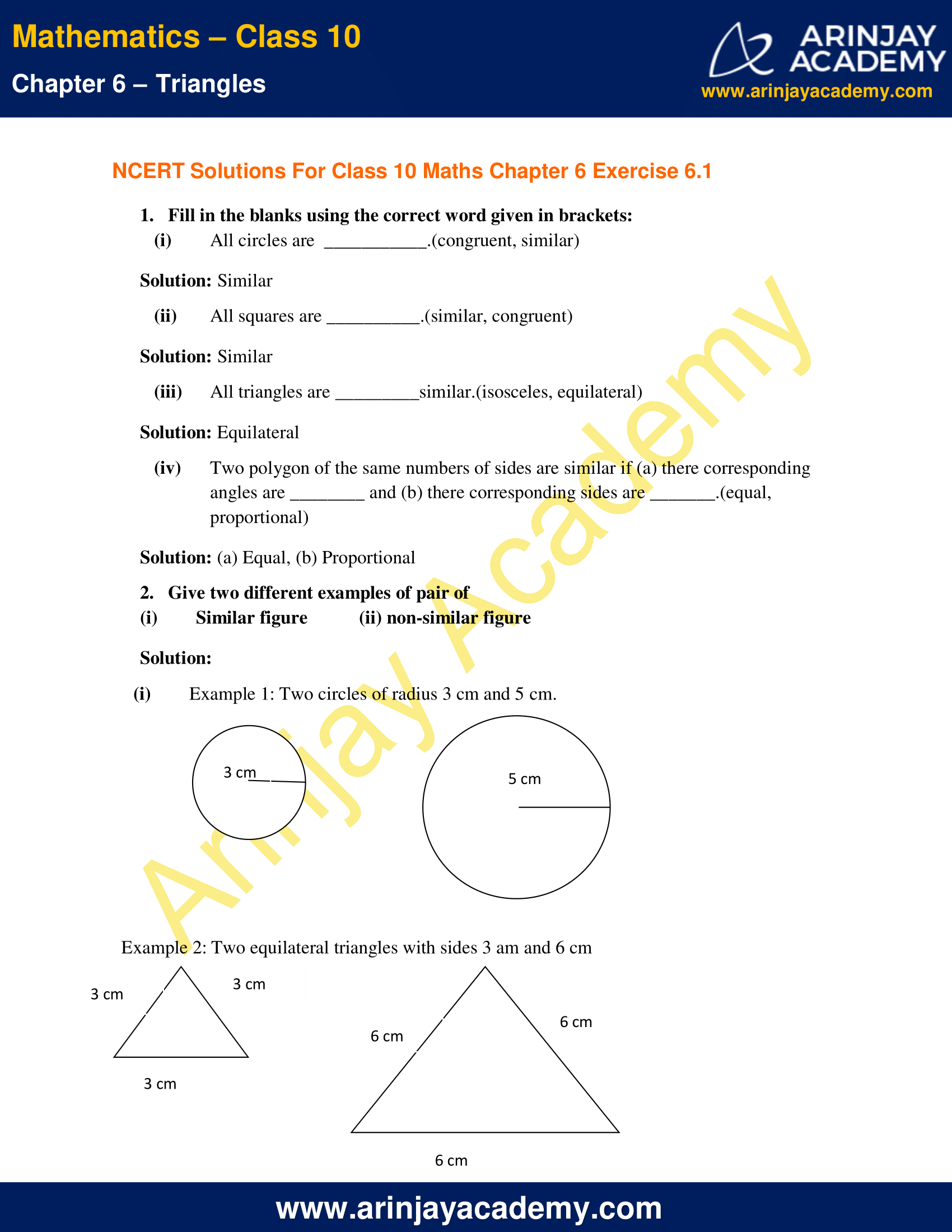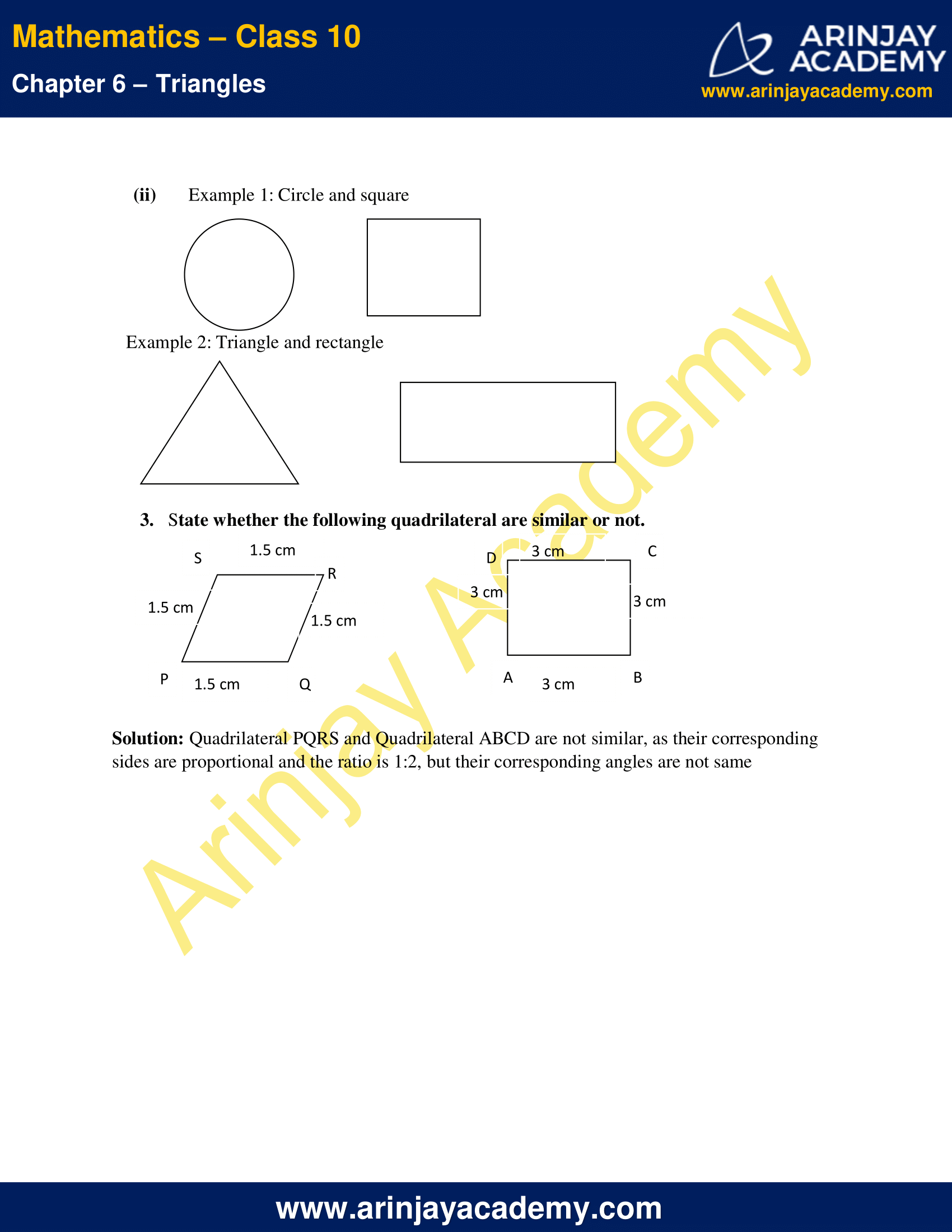NCERT Solutions For Class 10 Maths Chapter 6 Exercise 6.1 – Triangles

1. Fill in the blanks using the correct word given in brackets:

(i) All circles are (congruent, similar)
Solution: Similar

(ii)All squares are __________.(similar, congruent)
Solution: Similar

(iii) All triangles are _________similar.(isosceles, equilateral)
Solution: Equilateral

(iv) Two polygon of the same numbers of sides are similar if (a) there corresponding angles are ________ and (b) there corresponding sides are _______.(equal, proportional)
Solution: (a) Equal, (b) Proportional

2. Give two different examples of pair of

(i) Similar figure
(ii) non-similar figure

Solution:

(i) Example 1: Two circles of radius 3 cm and 5 cm.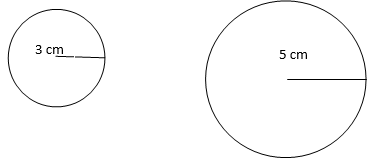Example 2: Two equilateral triangles with sides 3 am and 6 cm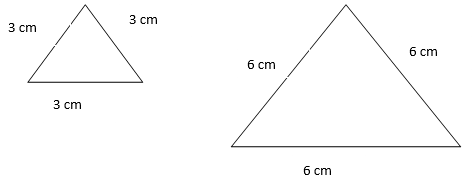(ii) Example 1: Circle and square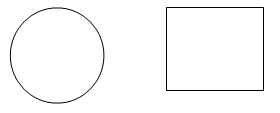Example 2: Triangle and rectangle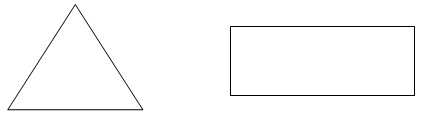3. State whether the following quadrilateral are similar or not.

Solution: Quadrilateral PQRS and Quadrilateral ABCD are not similar, as their corresponding sides are proportional and the ratio is 1:2, but their corresponding angles are not same.

NCERT Solutions for Class 10 Maths Chapter 6 Exercise 6.1 – Triangles, has been designed by the NCERT to test the knowledge of the student on the topic – Similar Figures